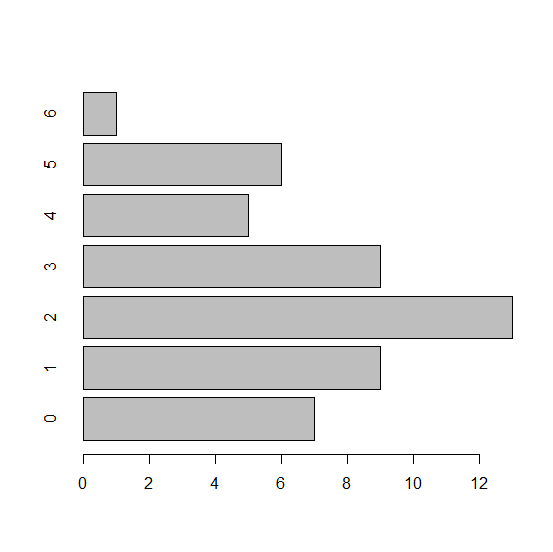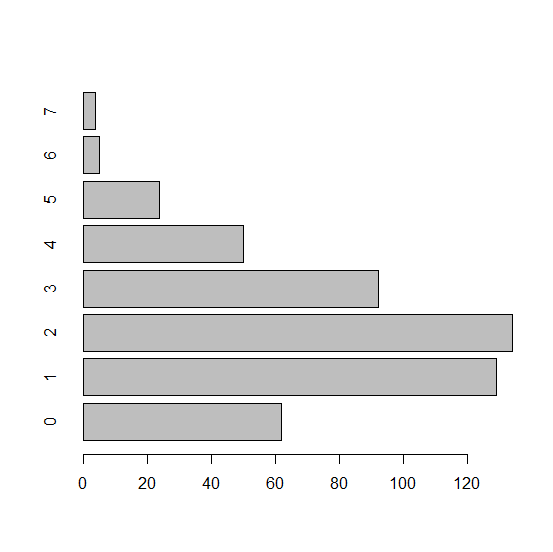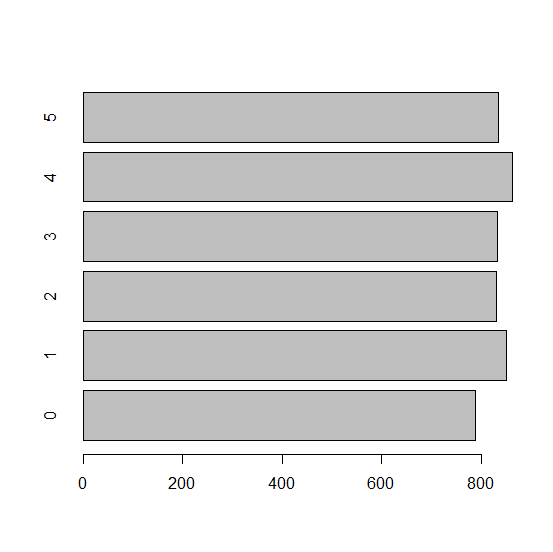# How to create a horizontal bar plot using barplot function in R?

To create a bar plot in base R, we can directly use barplot function but the table of frequencies should be passed inside this function. If we want to create the barplot in horizontal manner then horiz=TRUE argument must be added. For example, if we have a vector x that contains repeating values then the horizontal bar plot of x can be created by using barplot(table(x),horiz=TRUE).

## Example1

> x<-rpois(50,2)
> barplot(table(x),horiz=TRUE)

## Output## Example2

> y<-rpois(500,2)
> barplot(table(y),horiz=TRUE)

## Output## Example3

> z<-sample(0:5,5000,replace=TRUE)
> barplot(table(z),horiz=TRUE)

## Output